x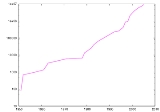Mersenne primeEncyclopedia
In mathematics
Mathematics
Mathematics is the study of quantity, space, structure, and change. Mathematicians seek out patterns and formulate new conjectures. Mathematicians resolve the truth or falsity of conjectures by mathematical proofs, which are arguments sufficient to convince other mathematicians of their validity...

, a Mersenne number, named after Marin Mersenne
Marin Mersenne
Marin Mersenne, Marin Mersennus or le Père Mersenne was a French theologian, philosopher, mathematician and music theorist, often referred to as the "father of acoustics"...

(a French monk who began the study of these numbers in the early 17th century), is a positive integer that is one less than a power of two
Power of two
In mathematics, a power of two means a number of the form 2n where n is an integer, i.e. the result of exponentiation with as base the number two and as exponent the integer n....

: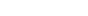Some definitions of Mersenne numbers require that the exponent p be prime, since the associated number must be composite if p is composite.

A Mersenne prime is a Mersenne number that is prime
Prime number
A prime number is a natural number greater than 1 that has no positive divisors other than 1 and itself. A natural number greater than 1 that is not a prime number is called a composite number. For example 5 is prime, as only 1 and 5 divide it, whereas 6 is composite, since it has the divisors 2...

. It is known that if 2p − 1 is prime then p is prime, so it makes no difference which Mersenne number definition is used. , 47 Mersenne primes are known. The largest known prime number (243,112,609 – 1
2^43112609 − 1
243112609 − 1 is the largest known Mersenne prime and the largest known prime number with 12,978,189 digits. Its discovery resulted from the Great Internet Mersenne Prime Search , and won its discoverers \$100,000 and a Cooperative Computing Award from the Electronic Frontier...

) is a Mersenne prime. Since 1997, all newly-found Mersenne primes have been discovered by the "Great Internet Mersenne Prime Search
Great Internet Mersenne Prime Search
The Great Internet Mersenne Prime Search is a collaborative project of volunteers who use freely available computer software to search for Mersenne prime numbers. The project was founded by George Woltman, who also wrote the software Prime95 and MPrime for the project...

" (GIMPS), a distributed computing
Distributed computing
Distributed computing is a field of computer science that studies distributed systems. A distributed system consists of multiple autonomous computers that communicate through a computer network. The computers interact with each other in order to achieve a common goal...

project on the Internet.

Many fundamental questions about Mersenne primes remain unresolved. It is not even known whether the set of Mersenne primes is finite. The Lenstra–Pomerance–Wagstaff conjecture asserts that, on the contrary, there are infinitely many Mersenne primes and predicts their order of growth
Asymptotic analysis
In mathematical analysis, asymptotic analysis is a method of describing limiting behavior. The methodology has applications across science. Examples are...

. It is also not known whether infinitely many Mersenne numbers with prime exponents are composite
Composite number
A composite number is a positive integer which has a positive divisor other than one or itself. In other words a composite number is any positive integer greater than one that is not a prime number....

, although this would follow from widely believed conjectures about prime numbers, for example, the infinitude of Sophie Germain prime
Sophie Germain prime
In number theory, a prime number p is a Sophie Germain prime if 2p + 1 is also prime. For example, 23 is a Sophie Germain prime because it is a prime and 2 × 23 + 1 = 47, and 47 is also a prime number...

s congruent
Congruence relation
In abstract algebra, a congruence relation is an equivalence relation on an algebraic structure that is compatible with the structure...

to 3 (mod 4
Modulo operation
In computing, the modulo operation finds the remainder of division of one number by another.Given two positive numbers, and , a modulo n can be thought of as the remainder, on division of a by n...

).

A basic theorem
Theorem
In mathematics, a theorem is a statement that has been proven on the basis of previously established statements, such as other theorems, and previously accepted statements, such as axioms...

about Mersenne numbers states that in order for Mp to be a Mersenne prime, the exponent p itself must be a prime number. This rules out primality for numbers such as M4 = 24 − 1 = 15: since the exponent 4 = 2×2 is composite
Composite number
A composite number is a positive integer which has a positive divisor other than one or itself. In other words a composite number is any positive integer greater than one that is not a prime number....

, the theorem predicts that 15 is also composite; indeed, 15 = 3×5. The three smallest Mersenne primes are
M2 = 3, M3 = 7, M5 = 31.

While it is true that only Mersenne numbers Mp, where p = 2, 3, 5, … could be prime, often Mp is not prime even for a prime exponent p. The smallest counterexample is the Mersenne number
M11 = 211 − 1 = 2047 = 23 × 89,

which is not prime, even though 11 is a prime number. The lack of an obvious rule to determine whether a given Mersenne number is prime makes the search for Mersenne primes an interesting task, which becomes difficult very quickly, since Mersenne numbers grow very rapidly. The Lucas–Lehmer primality test (LLT) is an efficient primality test
Primality test
A primality test is an algorithm for determining whether an input number is prime. Amongst other fields of mathematics, it is used for cryptography. Unlike integer factorization, primality tests do not generally give prime factors, only stating whether the input number is prime or not...

that greatly aids this task. The search for the largest known prime has somewhat of a cult following. Consequently, a lot of computer power has been expended searching for new Mersenne primes, much of which is now done using distributed computing
Distributed computing
Distributed computing is a field of computer science that studies distributed systems. A distributed system consists of multiple autonomous computers that communicate through a computer network. The computers interact with each other in order to achieve a common goal...

.

Mersenne primes are used in pseudorandom number generator
Pseudorandom number generator
A pseudorandom number generator , also known as a deterministic random bit generator , is an algorithm for generating a sequence of numbers that approximates the properties of random numbers...

s such as the Mersenne twister, Park–Miller random number generator
Park–Miller random number generator
The Lehmer random number generator , sometimes also referred to as the Park–Miller random number generator , is a variant of linear congruential generator that operates in multiplicative group of integers modulo n...

, Generalized Shift Register and Fibonacci RNG.

## Searching for Mersenne primes

The identity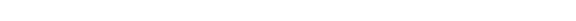shows that Mp can be prime only if p itself is prime, which simplifies the search for Mersenne primes considerably. The converse statement, namely that Mp is necessarily prime if p is prime, is false. The smallest counterexample is 211 − 1 = 2,047 = 23 × 89, a composite number
Composite number
A composite number is a positive integer which has a positive divisor other than one or itself. In other words a composite number is any positive integer greater than one that is not a prime number....

.

Fast algorithms for finding Mersenne primes are available, and the largest known prime numbers as of 2009 are Mersenne primes.

The first four Mersenne primes M2 = 3, M3 = 7, M5 = 31 and M7 = 127 were known in antiquity. The fifth, M13 = 8191, was discovered anonymously before 1461; the next two (M17 and M19) were found by Cataldi
Pietro Cataldi
Pietro Antonio Cataldi was an Italian mathematician. A citizen of Bologna, he taught mathematics and astronomy and also worked on military problems. His work included the development of continued fractions and a method for their representation. He was one of many mathematicians who attempted to...

in 1588. After nearly two centuries, M31 was verified to be prime by Euler
Leonhard Euler
Leonhard Euler was a pioneering Swiss mathematician and physicist. He made important discoveries in fields as diverse as infinitesimal calculus and graph theory. He also introduced much of the modern mathematical terminology and notation, particularly for mathematical analysis, such as the notion...

in 1772. The next (in historical, not numerical order) was M127, found by Lucas
Edouard Lucas
François Édouard Anatole Lucas was a French mathematician. Lucas is known for his study of the Fibonacci sequence. The related Lucas sequences and Lucas numbers are named after him.-Biography:...

in 1876, then M61 by Pervushin
Ivan Mikheevich Pervushin
Ivan Mikheevich Pervushin was an important Russian mathematician of the 2nd half of 19th century....

in 1883. Two more (M89 and M107) were found early in the 20th century, by Powers
R. E. Powers
Details of the life of R.E. Powers are little-known; however, he was apparently the first mathematician to demonstrate that the Mersenne number M107 = 2107 − 1 was indeed prime. This was published in his article Certain composite Mersenne's numbers in 1916. Sometimes, mathematical textbooks...

in 1911 and 1914, respectively.

The best method presently known for testing the primality of Mersenne numbers is the Lucas–Lehmer primality test. Specifically, it can be shown that for prime p > 2, Mp = 2p − 1 is prime if and only if Mp divides Sp−2, where S0 = 4 and, for k > 0,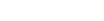The search for Mersenne primes was revolutionized by the introduction of the electronic digital computer. Alan Turing
Alan Turing
Alan Mathison Turing, OBE, FRS , was an English mathematician, logician, cryptanalyst, and computer scientist. He was highly influential in the development of computer science, providing a formalisation of the concepts of "algorithm" and "computation" with the Turing machine, which played a...

searched for them on the Manchester Mark 1
Manchester Mark 1
The Manchester Mark 1 was one of the earliest stored-program computers, developed at the Victoria University of Manchester from the Small-Scale Experimental Machine or "Baby" . It was also called the Manchester Automatic Digital Machine, or MADM...

in 1949. But the first successful identification of a Mersenne prime, M521, by this means was achieved at 10:00 P.M. on January 30, 1952 using the U.S. National Bureau of Standards Western Automatic Computer (SWAC)
SWAC (computer)
The SWAC was an early electronic digital computer built in 1950 by the U.S. National Bureau of Standards in Los Angeles, California. It was designed by Harry Huskey...

at the Institute for Numerical Analysis at the University of California, Los Angeles
University of California, Los Angeles
The University of California, Los Angeles is a public research university located in the Westwood neighborhood of Los Angeles, California, USA. It was founded in 1919 as the "Southern Branch" of the University of California and is the second oldest of the ten campuses...

, under the direction of Lehmer
Derrick Henry Lehmer
Derrick Henry "Dick" Lehmer was an American mathematician who refined Édouard Lucas' work in the 1930s and devised the Lucas–Lehmer test for Mersenne primes...

, with a computer search program written and run by Prof. R.M. Robinson
Raphael M. Robinson
Raphael Mitchel Robinson was an American mathematician.Born in National City, California, Robinson was the youngest of four children of a lawyer and a teacher. He was awarded the BA , MA , and Ph.D. , all in mathematics, and all from the University of California, Berkeley. His Ph.D...

. It was the first Mersenne prime to be identified in thirty-eight years; the next one, M607, was found by the computer a little less than two hours later. Three more — M1279, M2203, M2281 — were found by the same program in the next several months. M4253 is the first Mersenne prime that is titanic
Titanic prime
Titanic prime is a term coined by Samuel Yates in the 1980s, denoting a prime number of at least 1000 decimal digits. Few such primes were known then, but the required size is trivial for modern computers.The first 30 titanic primes are of the form:...

, M44497 is the first gigantic
Gigantic prime
A gigantic prime is a prime number with at least 10,000 decimal digits.The term appeared in Journal of Recreational Mathematics in the article "Collecting gigantic and titanic primes" by Samuel Yates...

, and M6,972,593 was the first megaprime
Megaprime
A megaprime is a prime number with at least one million decimal digits ., 35 megaprimes are known...

to be discovered, being a prime with at least 1,000,000 digits. All three were the first known prime of any kind of that size.

In September 2008, mathematicians at UCLA
University of California, Los Angeles
The University of California, Los Angeles is a public research university located in the Westwood neighborhood of Los Angeles, California, USA. It was founded in 1919 as the "Southern Branch" of the University of California and is the second oldest of the ten campuses...

participating in GIMPS won part of a \$100,000 prize from the Electronic Frontier Foundation
Electronic Frontier Foundation
The Electronic Frontier Foundation is an international non-profit digital rights advocacy and legal organization based in the United States...

for their discovery of a very nearly 13-million-digit Mersenne prime. The prize, finally confirmed in October 2009, is for the first known prime with at least 10 million digits. The prime was found on a Dell OptiPlex
Dell OptiPlex
Dell, Inc. targets its OptiPlex line of desktop computers for sale into the corporate, government and education markets. These systems typically contain Intel CPUs, beginning with the Pentium and with the Core i7 , although Dell sells some models with AMD CPUs as well...

745 on August 23, 2008. This is the eighth Mersenne prime discovered at UCLA.

On April 12, 2009, a GIMPS server log reported that a 47th Mersenne prime had possibly been found. This report was apparently overlooked until June 4, 2009. The find was verified on June 12, 2009. The prime is 242,643,801 − 1. Although it is chronologically the 47th Mersenne prime to be discovered, it is less than the largest known which was the 45th to be discovered.

1. If a and p are natural numbers such that ap − 1 is prime, then a = 2 or p = 1.
• Proof: Then so Thus However, is prime, so or In the former case, hence (which is a contradiction, as neither 1 nor 0 is prime) or In the latter case, or If however, which is not prime. Therefore,
2. If 2p − 1 is prime, then p is prime.
• Proof: suppose that p is composite, hence can be written with a and Then is prime, but and contradicting statement 1.
3. If p is an odd prime, then any prime q that divides 2p − 1 must be 1 plus a multiple of 2p. This holds even when 2p − 1 is prime.
• Examples: Example I: 25 − 1 = 31 is prime, and 31 = 1 + 3×2×5. Example II: 211 − 1 = 23×89, where 23 = 1 + 2×11, and 89 = 1 + 4×2×11.
• Proof: If q divides 2p − 1 then 2p ≡ 1 (mod q). By Fermat's Little Theorem
Fermat's little theorem
Fermat's little theorem states that if p is a prime number, then for any integer a, a p − a will be evenly divisible by p...

, 2(q − 1) ≡ 1 (mod q). Assume p and q − 1 are relatively prime, a similar application of Fermat's Little Theorem says that (q − 1)(p − 1) ≡ 1 (mod p). Thus there is a number x ≡ (q − 1)(p − 2) for which (q − 1)·x ≡ 1 (mod p), and therefore a number k for which (q − 1)·x − 1 = kp. Since 2(q − 1) ≡ 1 (mod q), raising both sides of the congruence
Modular arithmetic
In mathematics, modular arithmetic is a system of arithmetic for integers, where numbers "wrap around" after they reach a certain value—the modulus....

to the power x gives 2(q − 1)x ≡ 1, and since 2p ≡ 1 (mod q), raising both sides of the congruence to the power k gives 2kp ≡ 1. Thus 2(q − 1)x/2kp = 2(q − 1)x − kp ≡ 1 (mod q). But by definition, (q − 1)x − kp = 1, implying that 21 ≡ 1 (mod q); in other words, that q divides 1. Thus the initial assumption that p and q − 1 are relatively prime is untenable. Since p is prime q − 1 must be a multiple of p. Of course, if the number m = (q − 1)p is odd, then q will be even, since it is equal to mp + 1. But q is prime and cannot be equal to 2; therefore, m is even.
• Note: This fact provides a proof of the infinitude of primes distinct from Euclid's Theorem
Euclid's theorem
Euclid's theorem is a fundamental statement in number theory that asserts that there are infinitely many prime numbers. There are several well-known proofs of the theorem.-Euclid's proof:...

: if there were finitely many primes, with p being the largest, we reach an immediate contradiction since all primes dividing 2p − 1 must be larger than p.
4. If p is an odd prime, then any prime q that divides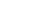must be congruent to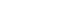.
• Proof: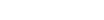, so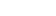is a square root of 2 modulo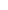. By quadratic reciprocity
In number theory, the law of quadratic reciprocity is a theorem about modular arithmetic which gives conditions for the solvability of quadratic equations modulo prime numbers...

, any prime modulo which 2 has a square root is congruent to.
5. A Mersenne prime cannot be a Wieferich prime
Wieferich prime
In number theory, a Wieferich prime is a prime number p such that p2 divides 2p − 1 − 1, therefore connecting these primes with Fermat's little theorem, which states that every odd prime p divides 2p − 1 − 1...

.
• Proof: We show if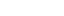is a Mersenne prime, then the congruence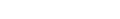does not satisfy. By Fermat's Little theorem,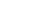. Now write,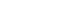. If the given congruence satisfies, then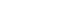,therefore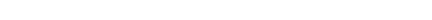. Hence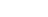,and therefore λ ≥ 2m − 1. This leads to p − 1  ≥ m(2m − 1), which is impossible since m ≥ 2.
6. A prime number divides at most one prime-exponent Mersenne number

## History

Mersenne primes were considered already by Euclid, who found a connection with the perfect number
Perfect number
In number theory, a perfect number is a positive integer that is equal to the sum of its proper positive divisors, that is, the sum of its positive divisors excluding the number itself . Equivalently, a perfect number is a number that is half the sum of all of its positive divisors i.e...

s. They are named after 17th-century French
France
The French Republic , The French Republic , The French Republic , (commonly known as France , is a unitary semi-presidential republic in Western Europe with several overseas territories and islands located on other continents and in the Indian, Pacific, and Atlantic oceans. Metropolitan France...

scholar Marin Mersenne
Marin Mersenne
Marin Mersenne, Marin Mersennus or le Père Mersenne was a French theologian, philosopher, mathematician and music theorist, often referred to as the "father of acoustics"...

, who compiled what was supposed to be a list of Mersenne primes with exponents up to 257. His list was largely incorrect, as Mersenne mistakenly included M67 and M257 (which are composite), and omitted M61, M89, and M107 (which are prime). Mersenne gave little indication how he came up with his list. A correct list of all Mersenne primes in this number range was completed and rigorously verified only about three centuries later.

## List of known Mersenne primes

The table below lists all known Mersenne primes :
# p Mp Mp digits Date of discovery Discoverer Method used
1 2 3 1 c. 500 BCE Ancient Greek
Ancient Greece
Ancient Greece is a civilization belonging to a period of Greek history that lasted from the Archaic period of the 8th to 6th centuries BC to the end of antiquity. Immediately following this period was the beginning of the Early Middle Ages and the Byzantine era. Included in Ancient Greece is the...

mathematicians
2 3 7 1 c. 500 BCE Ancient Greek mathematicians
3 5 31
31 (number)
31 is the natural number following 30 and preceding 32.- In mathematics :Thirty-one is the third Mersenne prime as well as the fourth primorial prime, and together with twenty-nine, another primorial prime, it comprises a twin prime. As a Mersenne prime, 31 is related to the perfect number 496,...

2 c. 275 BCE Ancient Greek mathematicians
4 7 127
127 (number)
127 is the natural number following 126 and preceding 128.- In mathematics :*As a Mersenne prime, 127 is related to the perfect number 8128. 127 is also an exponent for the Mersenne prime 2127 - 1, making 127 a double Mersenne prime...

3 c. 275 BCE Ancient Greek mathematicians
5 13 8191 4 1456 Anonymous Trial division
Trial division
Trial division is the most laborious but easiest to understand of the integer factorization algorithms. Its ease of implementation makes it a viable integer factorization option for devices with little available memory, such as graphing calculators....

6 17 131071 6 1588 Pietro Cataldi
Pietro Cataldi
Pietro Antonio Cataldi was an Italian mathematician. A citizen of Bologna, he taught mathematics and astronomy and also worked on military problems. His work included the development of continued fractions and a method for their representation. He was one of many mathematicians who attempted to...

Trial division
7 19 524287 6 1588 Pietro Cataldi Trial division
8 31 2147483647
2147483647
The number 2,147,483,647 is the eighth Mersenne prime, equal to 231 − 1. It is one of only four known double Mersenne primes....

10 1772 Leonhard Euler
Leonhard Euler
Leonhard Euler was a pioneering Swiss mathematician and physicist. He made important discoveries in fields as diverse as infinitesimal calculus and graph theory. He also introduced much of the modern mathematical terminology and notation, particularly for mathematical analysis, such as the notion...

Enhanced trial division
9 61 2305843009213693951 19 1883 I. M. Pervushin
Ivan Mikheevich Pervushin
Ivan Mikheevich Pervushin was an important Russian mathematician of the 2nd half of 19th century....

Lucas sequence
Lucas sequence
In mathematics, the Lucas sequences Un and Vn are certain integer sequences that satisfy the recurrence relationwhere P and Q are fixed integers...

s
10 89 618970019…449562111 27 1911 June R. E. Powers
R. E. Powers
Details of the life of R.E. Powers are little-known; however, he was apparently the first mathematician to demonstrate that the Mersenne number M107 = 2107 − 1 was indeed prime. This was published in his article Certain composite Mersenne's numbers in 1916. Sometimes, mathematical textbooks...

Lucas sequences
11 107 162259276…010288127 33 1914 June 11 R. E. Powers
R. E. Powers
Details of the life of R.E. Powers are little-known; however, he was apparently the first mathematician to demonstrate that the Mersenne number M107 = 2107 − 1 was indeed prime. This was published in his article Certain composite Mersenne's numbers in 1916. Sometimes, mathematical textbooks...

Lucas sequences
12 127 170141183…884105727 39 1876 January 10 Édouard Lucas
Edouard Lucas
François Édouard Anatole Lucas was a French mathematician. Lucas is known for his study of the Fibonacci sequence. The related Lucas sequences and Lucas numbers are named after him.-Biography:...

Lucas sequences
13 521 686479766…115057151 157 1952 January 30 Raphael M. Robinson
Raphael M. Robinson
Raphael Mitchel Robinson was an American mathematician.Born in National City, California, Robinson was the youngest of four children of a lawyer and a teacher. He was awarded the BA , MA , and Ph.D. , all in mathematics, and all from the University of California, Berkeley. His Ph.D...

LLT / SWAC
SWAC (computer)
The SWAC was an early electronic digital computer built in 1950 by the U.S. National Bureau of Standards in Los Angeles, California. It was designed by Harry Huskey...

14 607 531137992…031728127 183 1952 January 30 Raphael M. Robinson LLT / SWAC
15 1,279 104079321…168729087 386 1952 June 25 Raphael M. Robinson LLT / SWAC
16 2,203 147597991…697771007 664 1952 October 7 Raphael M. Robinson LLT / SWAC
17 2,281 446087557…132836351 687 1952 October 9 Raphael M. Robinson LLT / SWAC
18 3,217 259117086…909315071 969 1957 September 8 Hans Riesel
Hans Riesel
Hans Ivar Riesel is a Swedish mathematician who discovered the 18th known Mersenne prime in 1957, using the computer BESK. This prime is 23217-1, which consists of 969 digits. He held the record for the largest known prime from 1957 to 1961, when Alexander Hurwitz discovered a larger one. Riesel...

LLT / BESK
BESK
BESK was Sweden's first electronic computer, using vacuum tubes instead of relays. It was developed by Matematikmaskinnämnden and during a short time it was the fastest computer in the world. The computer was completed in 1953 and in use until 1966...

19 4,253 190797007…350484991 1,281 1961 November 3 Alexander Hurwitz LLT / IBM 7090
IBM 7090
The IBM 7090 was a second-generation transistorized version of the earlier IBM 709 vacuum tube mainframe computers and was designed for "large-scale scientific and technological applications". The 7090 was the third member of the IBM 700/7000 series scientific computers. The first 7090 installation...

20 4,423 285542542…608580607 1,332 1961 November 3 Alexander Hurwitz LLT / IBM 7090
21 9,689 478220278…225754111 2,917 1963 May 11 Donald B. Gillies
Donald B. Gillies
Donald Bruce Gillies was a Canadian mathematician and computer scientist, known for his work in game theory, computer design, and minicomputer programming environments.- Education :...

LLT / ILLIAC II
ILLIAC II
The ILLIAC II was a revolutionary super-computer built by the University of Illinois that became operational in 1962.-Description:The concept, proposed in 1958, pioneered Emitter-coupled logic circuitry, pipelining, and transistor memory with a design goal of 100x speedup compared to ILLIAC...

22 9,941 346088282…789463551 2,993 1963 May 16 Donald B. Gillies LLT / ILLIAC II
23 11,213 281411201…696392191 3,376 1963 June 2 Donald B. Gillies LLT / ILLIAC II
24 19,937 431542479…968041471 6,002 1971 March 4 Bryant Tuckerman
Bryant Tuckerman
Louis Bryant Tuckerman, III was an American mathematician, born in Lincoln, Nebraska. He was a member of the team that developed the Data Encryption Standard ....

LLT / IBM 360/91
25 21,701 448679166…511882751 6,533 1978 October 30 Landon Curt Noll
Landon Curt Noll
Landon Curt Noll is an American computer scientist, co-discoverer of the 25th Mersenne prime and discoverer of the 26th, which he found while still enrolled in high school and concurrently at Cal State Hayward....

& Laura Nickel
LLT / CDC Cyber
CDC Cyber
The CDC Cyber range of mainframe-class supercomputers were the primary products of Control Data Corporation during the 1970s and 1980s. In their day, they were the computer architecture of choice for scientific and mathematically intensive computing...

174
26 23,209 402874115…779264511 6,987 1979 February 9 Landon Curt Noll LLT / CDC Cyber 174
27 44,497 854509824…011228671 13,395 1979 April 8 Harry Lewis Nelson
Harry Lewis Nelson
Harry Lewis Nelson is an American mathematician and computer programmer. He was a member of the team that won the World Computer Chess Championship in 1983 and 1986, and was a co-discoverer of the 27th Mersenne prime in 1979 . He also served as editor of the Journal of Recreational Mathematics for...

& David Slowinski
David Slowinski
David Slowinski is a mathematician involved in prime numbers. His career highlights have included the discovery of several of the largest known Mersenne primes:1* 244497−1 on April 8 1979* 286243−1 on September 25 1982...

LLT / Cray 1
28 86,243 536927995…433438207 25,962 1982 September 25 David Slowinski LLT / Cray 1
29 110,503 521928313…465515007 33,265 1988 January 28 Walter Colquitt & Luke Welsh LLT / NEC SX-2
30 132,049 512740276…730061311 39,751 1983 September 19 David Slowinski LLT / Cray X-MP
Cray X-MP
The Cray X-MP was a supercomputer designed, built and sold by Cray Research. It was announced in 1982 as the "cleaned up" successor to the 1975 Cray-1, and was the world's fastest computer from 1983 to 1985...

31 216,091 746093103…815528447 65,050 1985 September 1 David Slowinski LLT / Cray X-MP/24
32 756,839 174135906…544677887 227,832 1992 February 19 David Slowinski & Paul Gage
Paul Gage
Paul Gage is a research computer scientist who works at Cray Supercomputers. He is most well known for collaborating with David Slowinski on an algorithm that is credited with the discovery of several of the largest Mersenne primes: 2756839−1 in 1992, 2859433−1 in 1994, and 21257787−1 in...

LLT / Maple
Maple (software)
Maple is a general-purpose commercial computer algebra system. It was first developed in 1980 by the Symbolic Computation Group at the University of Waterloo in Waterloo, Ontario, Canada....

on Harwell Lab Cray-2
Cray-2
The Cray-2 was a four-processor ECL vector supercomputer made by Cray Research starting in 1985. It was the fastest machine in the world when it was released, replacing the Cray Research X-MP designed by Steve Chen in that spot...

33 859,433 129498125…500142591 258,716 1994 January 4 David Slowinski & Paul Gage LLT / Cray C90
Cray C90
The Cray C90 series was a vector processor supercomputer launched by Cray Research in 1991. The C90 was a development of the Cray Y-MP architecture. Compared to the Y-MP, the C90 processor had a dual vector pipeline and a faster 4.1 ns clock cycle , which together gave three times the...

34 1,257,787 412245773…089366527 378,632 1996 September 3 David Slowinski & Paul Gage LLT / Cray T94
Cray T90
The Cray T90 series was the last of a line of vector processing supercomputers manufactured by Cray Research, Inc, superseding the Cray C90 series...

35 1,398,269 814717564…451315711 420,921 1996 November 13 GIMPS
Great Internet Mersenne Prime Search
The Great Internet Mersenne Prime Search is a collaborative project of volunteers who use freely available computer software to search for Mersenne prime numbers. The project was founded by George Woltman, who also wrote the software Prime95 and MPrime for the project...

/ Joel Armengaud
LLT / Prime95
Prime95
Prime95 is the name of the Microsoft Windows-based software application written by George Woltman that is used by GIMPS, a distributed computing project dedicated to finding new Mersenne prime numbers....

on 90 MHz Pentium
Pentium
The original Pentium microprocessor was introduced on March 22, 1993. Its microarchitecture, deemed P5, was Intel's fifth-generation and first superscalar x86 microarchitecture. As a direct extension of the 80486 architecture, it included dual integer pipelines, a faster FPU, wider data bus,...

PC
36 2,976,221 623340076…729201151 895,932 1997 August 24 GIMPS / Gordon Spence LLT / Prime95 on 100 MHz Pentium PC
37 3,021,377 127411683…024694271 909,526 1998 January 27 GIMPS / Roland Clarkson LLT / Prime95 on 200 MHz Pentium PC
38 6,972,593 437075744…924193791 2,098,960 1999 June 1 GIMPS / Nayan Hajratwala LLT / Prime95 on 350 MHz IBM Aptiva
IBM Aptiva
The IBM Aptiva personal computer was introduced in September 1994 as the replacement for the IBM PS/1. The first Aptiva models were based on the Intel 80486 CPU with later models using the Pentium and AMD CPUs. All systems were developed in-house except for the later E series which was developed by...

39 13,466,917 924947738…256259071 4,053,946 2001 November 14 GIMPS / Michael Cameron LLT / Prime95 on 800 MHz Athlon T-Bird
40 20,996,011 125976895…855682047 6,320,430 2003 November 17 GIMPS / Michael Shafer LLT / Prime95 on 2 GHz Dell Dimension
Dell Dimension
Dell Dimension was a line of home desktop computers manufactured by Dell Inc. As of June 26, 2007, Dell replaced the low-end Dimension with the Inspiron line of desktop computers, later offering higher-end consumer machines under the Studio line in 2008. The Dimension is now sold to non-American...

41 24,036,583 299410429…733969407 7,235,733 2004 May 15 GIMPS / Josh Findley LLT / Prime95 on 2.4 GHz Pentium 4
Pentium 4
Pentium 4 was a line of single-core desktop and laptop central processing units , introduced by Intel on November 20, 2000 and shipped through August 8, 2008. They had a 7th-generation x86 microarchitecture, called NetBurst, which was the company's first all-new design since the introduction of the...

PC
42 25,964,951 122164630…577077247 7,816,230 2005 February 18 GIMPS / Martin Nowak LLT / Prime95 on 2.4 GHz Pentium 4
Pentium 4
Pentium 4 was a line of single-core desktop and laptop central processing units , introduced by Intel on November 20, 2000 and shipped through August 8, 2008. They had a 7th-generation x86 microarchitecture, called NetBurst, which was the company's first all-new design since the introduction of the...

PC
43 30,402,457 315416475…652943871 9,152,052 2005 December 15 GIMPS / Curtis Cooper
Curtis Cooper (mathematician)
Curtis Niles Cooper is an American mathematician. He currently is a professor at the University of Central Missouri, in the Department of Mathematics and Computer Science....

& Steven Boone
LLT / Prime95 on 2 GHz Pentium 4 PC
44 32,582,657 124575026…053967871 9,808,358 2006 September 4 GIMPS / Curtis Cooper & Steven Boone LLT / Prime95 on 3 GHz Pentium 4 PC
45 37,156,667 202254406…308220927 11,185,272 2008 September 6 GIMPS / Hans-Michael Elvenich LLT / Prime95 on 2.83 GHz Core 2 Duo PC
46 42,643,801 169873516…562314751 12,837,064 2009 April 12 GIMPS / Odd M. Strindmo LLT / Prime95 on 3 GHz Core 2 PC
47 43,112,609 316470269…697152511 12,978,189 2008 August 23 GIMPS / Edson Smith LLT / Prime95 on Dell Optiplex
Dell OptiPlex
Dell, Inc. targets its OptiPlex line of desktop computers for sale into the corporate, government and education markets. These systems typically contain Intel CPUs, beginning with the Pentium and with the Core i7 , although Dell sells some models with AMD CPUs as well...

745

It is not known whether any undiscovered Mersenne primes exist between the 41st (M24,036,583) and the 47th (M43,112,609) on this chart; the ranking is therefore provisional. Primes are not always discovered in increasing order. For example, the 29th Mersenne prime was discovered after the 30th and the 31st. Similarly, the current record holder was followed by two smaller Mersenne primes, first 2 weeks later and then 8 months later.

M42,643,801 was first found by a machine on April 12, 2009; however, no human took notice of this fact until June 4. Thus, either April 12 or June 4 may be considered the 'discovery' date. The discoverer, Strindmo, apparently used the alias Stig M. Valstad.

To help visualize the size of the 47th known Mersenne prime, it would require 3,461 pages to display the number in base 10 with 75 digits per line and 50 lines per page.

The largest known Mersenne prime (243,112,609 − 1) is also the largest known prime number, and was the first discovered prime number with more than 10 million base-10 digits.

In modern times, the largest known prime has almost always been a Mersenne prime.

## 2305843009213693951

The number
Number
A number is a mathematical object used to count and measure. In mathematics, the definition of number has been extended over the years to include such numbers as zero, negative numbers, rational numbers, irrational numbers, and complex numbers....

2305843009213693951 is the ninth Mersenne prime and is equal to 261 − 1. It was determined to be prime in 1883 by Ivan Mikheevich Pervushin
Ivan Mikheevich Pervushin
Ivan Mikheevich Pervushin was an important Russian mathematician of the 2nd half of 19th century....

and for this reason it is sometimes called Pervushin's number. At the time of Pervushin's proof it was the second-largest known prime number, (Édouard Lucas
Edouard Lucas
François Édouard Anatole Lucas was a French mathematician. Lucas is known for his study of the Fibonacci sequence. The related Lucas sequences and Lucas numbers are named after him.-Biography:...

having shown earlier that 2127 − 1 is also prime), and it remained so until 1911.

## Factorization of Mersenne numbers

The factorization of a prime number is by definition the number itself. This section is about composite numbers. Mersenne numbers are very good test cases for the special number field sieve
Special number field sieve
In number theory, a branch of mathematics, the special number field sieve is a special-purpose integer factorization algorithm. The general number field sieve was derived from it....

algorithm, so often the largest number factorized with this algorithm has been a Mersenne number. , 21039 − 1 is the record-holder, after a calculation taking about a year on a couple of hundred computers, mostly at NTT
Nippon Telegraph and Telephone
, commonly known as NTT, is a Japanese telecommunications company headquartered in Tokyo, Japan. Ranked the 31st in Fortune Global 500, NTT is the largest telecommunications company in Asia, and the second-largest in the world in terms of revenue....

in Japan and at EPFL
École polytechnique fédérale de Lausanne
The École polytechnique fédérale de Lausanne is one of the two Swiss Federal Institutes of Technology and is located in Lausanne, Switzerland.The school was founded by the Swiss Federal Government with the stated mission to:...

in Switzerland. See integer factorization records
Integer factorization records
Integer factorization is the process of determining which prime numbers divide a given positive integer. Doing this quickly has applications in cryptography...

for links to more information. The special number field sieve can factorize numbers with more than one large factor. If a number has only one very large factor then other algorithms can factorize larger numbers by first finding small factors and then making a primality test
Primality test
A primality test is an algorithm for determining whether an input number is prime. Amongst other fields of mathematics, it is used for cryptography. Unlike integer factorization, primality tests do not generally give prime factors, only stating whether the input number is prime or not...

on the cofactor. , the composite Mersenne number with largest proven prime factors is 220887 − 1, which is known to have a factor p with 6229 digits that was proven prime with ECPP
Elliptic curve primality proving
Elliptic Curve Primality Proving is a method based on elliptic curves to prove the primality of a number . It is a general-purpose algorithm, meaning it does not depend on the number being of a special form...

. The largest with probable prime
Probable prime
In number theory, a probable prime is an integer that satisfies a specific condition also satisfied by all prime numbers. Different types of probable primes have different specific conditions...

factors allowed is 21168183 − 1 = 54763676838381762583 × q, where q is a probable prime.

## Perfect numbers

Mersenne primes are interesting to many for their connection to perfect number
Perfect number
In number theory, a perfect number is a positive integer that is equal to the sum of its proper positive divisors, that is, the sum of its positive divisors excluding the number itself . Equivalently, a perfect number is a number that is half the sum of all of its positive divisors i.e...

s. In the 4th century BCE, Euclid
Euclid
Euclid , fl. 300 BC, also known as Euclid of Alexandria, was a Greek mathematician, often referred to as the "Father of Geometry". He was active in Alexandria during the reign of Ptolemy I...

demonstrated that if Mp is a Mersenne prime then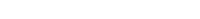is an even perfect number (Elements ix.36)—which is also the Mpth triangular number
Triangular number
A triangular number or triangle number numbers the objects that can form an equilateral triangle, as in the diagram on the right. The nth triangle number is the number of dots in a triangle with n dots on a side; it is the sum of the n natural numbers from 1 to n...

and the 2p−1th hexagonal number
Hexagonal number
A hexagonal number is a figurate number. The nth hexagonal number will be the number of points in a hexagon with n regularly spaced points on a side.The formula for the nth hexagonal number...

. In the 18th century, Leonhard Euler
Leonhard Euler
Leonhard Euler was a pioneering Swiss mathematician and physicist. He made important discoveries in fields as diverse as infinitesimal calculus and graph theory. He also introduced much of the modern mathematical terminology and notation, particularly for mathematical analysis, such as the notion...

proved that, conversely, all even perfect numbers have this form. It is unknown whether there are any odd perfect numbers, but it appears unlikely.

## Generalization

The binary
Binary numeral system
The binary numeral system, or base-2 number system, represents numeric values using two symbols, 0 and 1. More specifically, the usual base-2 system is a positional notation with a radix of 2...

representation of 2p − 1 is the digit 1 repeated p times, for example, 25 − 1 = 111112 in the binary notation. A Mersenne number is therefore a repunit
Repunit
In recreational mathematics, a repunit is a number like 11, 111, or 1111 that contains only the digit 1. The term stands for repeated unit and was coined in 1966 by Albert H. Beiler...

in base 2, and Mersenne primes are the base 2 repunit primes.

The base 2 representation of a Mersenne number shows the factorization pattern for composite exponent. For example: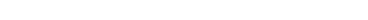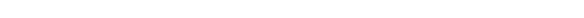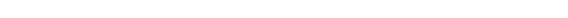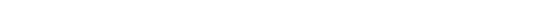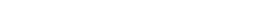## Mersenne numbers in nature and elsewhere

In computer science, unsigned
Signedness
In computing, signedness is a property of data types representing numbers in computer programs. A numeric variable is signed if it can represent both positive and negative numbers, and unsigned if it can only represent non-negative numbers .As signed numbers can represent negative numbers, they...

p-bit (or signed
Signed number representations
In computing, signed number representations are required to encode negative numbers in binary number systems.In mathematics, negative numbers in any base are represented by prefixing them with a − sign. However, in computer hardware, numbers are represented in binary only without extra...

p+1-bit) integers
Integer (computer science)
In computer science, an integer is a datum of integral data type, a data type which represents some finite subset of the mathematical integers. Integral data types may be of different sizes and may or may not be allowed to contain negative values....

can be used to express numbers up to Mp.

In the mathematical problem Tower of Hanoi
Tower of Hanoi
The Tower of Hanoi or Towers of Hanoi, also called the Tower of Brahma or Towers of Brahma, is a mathematical game or puzzle. It consists of three rods, and a number of disks of different sizes which can slide onto any rod...

, solving a puzzle with a p-disc tower requires at least Mp steps.

The asteroid
Asteroid
Asteroids are a class of small Solar System bodies in orbit around the Sun. They have also been called planetoids, especially the larger ones...

with minor planet
Minor planet
An asteroid group or minor-planet group is a population of minor planets that have a share broadly similar orbits. Members are generally unrelated to each other, unlike in an asteroid family, which often results from the break-up of a single asteroid...

number 8191 is named 8191 Mersenne
8191 Mersenne
8191 Mersenne is an asteroid discovered 1993 July 20 by Eric Walter Elst at La Silla Observatory, according to http://www.angelfire.com/id/ericelst/Citapla.html. It was named after Marin Mersenne because 8191, the number of the object discovered, is sequentially the fifth Mersenne prime.- External...

after Marin Mersenne, because 8191 is a Mersenne prime (3 Juno
3 Juno
Juno , formal designation 3 Juno in the Minor Planet Center catalogue system, was the third asteroid to be discovered and is one of the larger main-belt asteroids, being one of the two largest stony asteroids, along with 15 Eunomia. Juno is estimated to contain 1% of the total mass of the asteroid...

, 7 Iris
7 Iris
7 Iris is a large main-belt asteroid. Among the S-type asteroids, it ranks fifth in geometric mean diameter after Eunomia, Juno, Amphitrite and Herculina....

, 31 Euphrosyne
31 Euphrosyne
31 Euphrosyne is one of the largest main-belt asteroids, discovered by James Ferguson on September 1, 1854. It was the first asteroid found from North America. It is named after Euphrosyne, one of the Charites in Greek mythology....

and 127 Johanna
127 Johanna
127 Johanna is a large main-belt asteroid. It has a very dark surface and is probably a primitive composition of carbonates. It was discovered by P. M. Henry on November 5, 1872, and is believed to be named after Joan of Arc.-References:...

having been discovered and named during the nineteenth century).